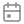# 10 Must-Know Excel Tips for Every SalespersonDec 18 2019## Top excel data analysis functions you need to know

As a sales executive, maintaining sales lead database in excel sheets is a big task. You experience the agony of selecting or choosing formulas or applying those functions to analyze your data sets. If the formulas are incorrect, the output will also be wrong. And if the functions are too complicated, you finally give up on the excel sheet.

There are many functions and formulas in Excel, and it would be time-consuming trying to match the right formula or function to obtain the right kind of data. Here are the top 10 functions to improve your capability to analyze data and achieve your sales goals.

Let’s get to it. Here are Top 10 excel tips to ease your work:

1. Use PIVOT TABLES:  Pivot tables are used to sort, count, total or average data stored in one massive spreadsheet. You can display the data in a new sheet or in the same, however, you want. For example, if you’re going to look only at sales figures for specific countries, product lines, or marketing channels then you need to ensure the data is clean.

Here is the image:• Click Insert-> Pivot table
• Select the table/ range to convert into a pivot table
• Choose location/sheet or where you want the Pivot Table report to place
• New Worksheet
• Existing Worksheet
• Click OKPivot Table appears on your right-hand side of the excel sheet

Select the field you want to find values and drag the fields between the selected areas displayed below-

• Report Filter: Allows only look at specific rows in your dataset
• Row Labels: Can be rows in your dataset
• Value: Allows you to look at your data differently. Such as you can sum, count, average, max, min, count numbers, etc. and can do a few more manipulations with your data to get the desired dataset. By default, when you drag any field to find the value, it always does a sum up the number present in the columns or rows selected.

You will be able to view the data now2. Use FILTERS: When you have an extensive database and want to look up for a specific product column or a particular row, then applying Filters give you the data that you are looking for.

• It allows you to trim down your data to only look at specific rows at one time.
• It can be added to each column in your data –from there; you can select which cells to view
• To add filter in your sheet- Click Data tab-> Select the row -> Click filters

3. Use VLOOKUP function:  If you have two sets of data on two different spreadsheets and you want to combine into a single spreadsheet, then the VLOOKUP function is used. In this function, there are several variables that you need to understand. The function is true and obtains results when you want to combine the information in Sheet 1 and Sheet 2 onto Sheet 1.The formula: =VLOOKUP(lookup value, table , column number, [range lookup])

• Note- Range lookup value is either TRUE or FALSE

5. Use COUNTIF: This function helps to make Excel count- words or numbers in any range of cells you want. COUNTIF is powerful because you can put the limitless criteria.

Formula: =COUNTIF(RANGE,CRITERIA)

For example, if you want to find out the count of the particular team name from the list.6. Use ABSOLUTE REFERENCE: Use dollar sign to keep one cell’s formula the same regardless of where it moves. Let’s take an example - if you want to change the relative formula (=B5+F5) into a perfect formula, then precede the row and column values by dollar signs, like this: (=\$B\$5+\$F\$5).

7. Use FLASH FILL: This function is useful to create email ID or save data in the manner you want to have. For example, you have two columns of names – First name and Last name. Now, you need to construct email addresses from them all.

• Just do for the first row
• Select the rows to generate emails-
• Go to Data tab-> click Flash Fill8. Use SLICERS- PivotTable slicers: To add a slicer to your PivotTable-> Insert tab- > Filters. This function will help you to show certain and hide certain data. It offers nice user-friendly buttons to enhance the user experience.

9. Use CHARTS: Charts are the best function to represent your data. There are more than 20 chart types, which include- Bar, Column, Pie, Line, and Scatter charts. These most effective ways to represent your data analysis.

To find chart-> Insert tab-> Charts sections

10. Use SUMPRODUCT: This is a great function to calculate the average sum of the rows you want. It helps to multiply one range of values by its corresponding row counterparts. For example, we calculate the average selling price of our ten products by using sumproduct to Price by Quantity, then divide by the total quantity sold.

Formula: =SUMPRODUCT(RANGE1,RANGE2)/SELECT CELL